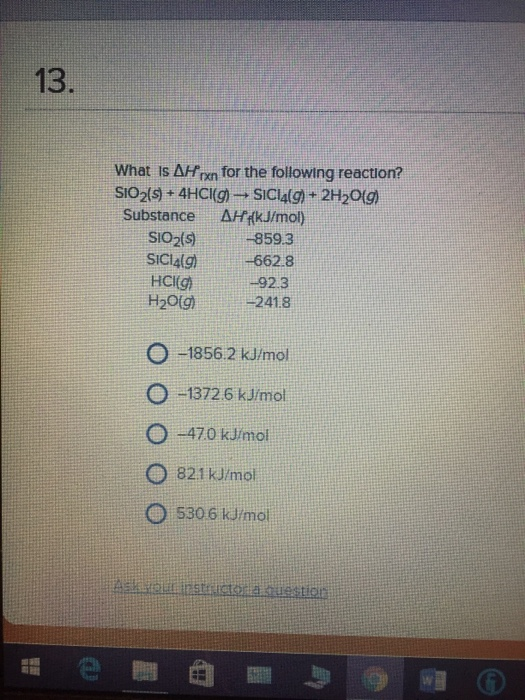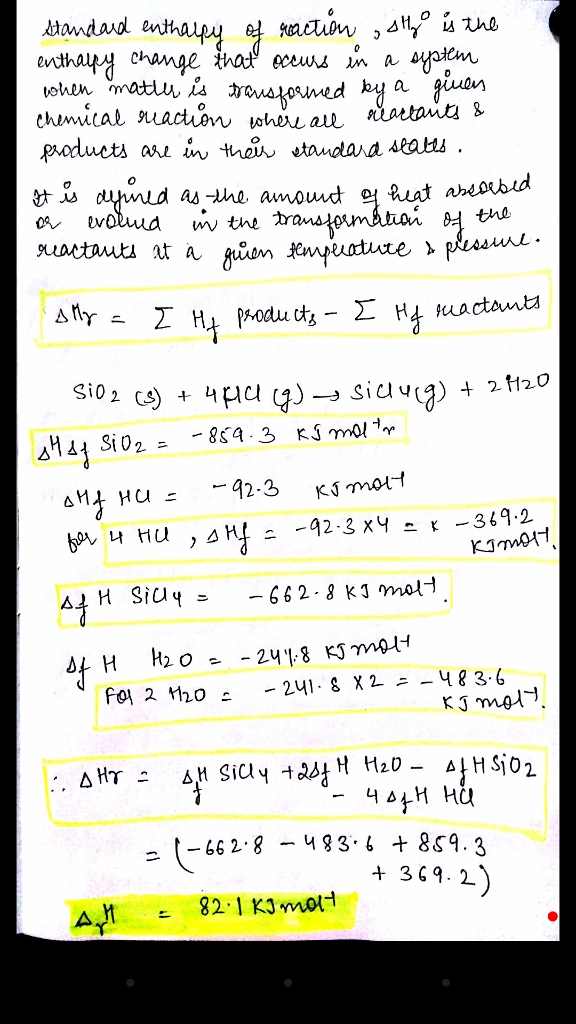# Question & Answer: What is Delta H_rnx degree for the following reaction? SiO2(S) + 4 HCI(g) rightarrow…..What is Delta H_rnx degree for the following reaction? SiO2(S) + 4 HCI(g) rightarrow SICI_4(g) + 2H_2O(g) Substance Delta H_f degree(kJ/mol) SiO_2(s) -859.3 SICI_4(g) -662.8 HCl(g) -92.3 H_2O(g) -241.8 -1856.2 kJ/mol -1372.6 kJ/mol -470 kJ/mol 82.1 kJ/mol 530.6 kJ/mol